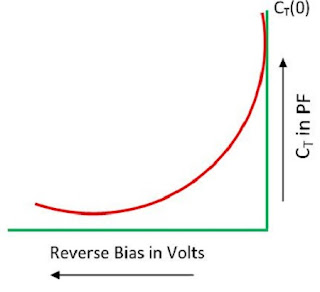Pages

Characteristics of varactor diode

The below graph illustrates the relation between the magnitude of reverse bias voltage p-type and n-type layers of a varactor diode, and the magnitude of junction capacitance. They are voltage controlled capacitor and sometimes known as varicap diodes although the word varactor is widely used.

Variable capacitance effect is shown by a normal diode, but the varactor diode is preferred for providing required capacitance changes. The diodes are uniquely optimized and also manufactured such that they enable high range changes in the capacitance device. A varactor diode is categorized based on the properties of a diode junction.

The characteristics of the varactor diode have the following:
• These diodes are significantly generated less noise compared to other diodes
• The cost of these diodes is available at lower or more reliable also
• These diodes are very small in size and very lightweight compared to other diodes
• There is no use when it is operated in forwarding bias
• In reverse bias mode, this diode enhanced the capacitance as shown in the graph belowCharacteristics of a varactor diode
The below graph illustrates the relation between the magnitude of reverse bias voltage p-type and n-type layers of a varactor diode, and the magnitude of junction capacitance. They are voltage controlled capacitor and sometimes known as varicap diodes although the word varactor is widely used.

Variable capacitance effect is shown by a normal diode, but the varactor diode is preferred for providing required capacitance changes. The diodes are uniquely optimized and also manufactured such that they enable high range changes in the capacitance device. A varactor diode is categorized based on the properties of a diode junction.

The characteristics of the varactor diode have the following:
• These diodes are significantly generated less noise compared to other diodes
• The cost of these diodes is available at lower or more reliable also
• These diodes are very small in size and very lightweight compared to other diodes
• There is no use when it is operated in forwarding bias
• In reverse bias mode, this diode enhanced the capacitance as shown in the graph belowCharacteristics of a varactor diode